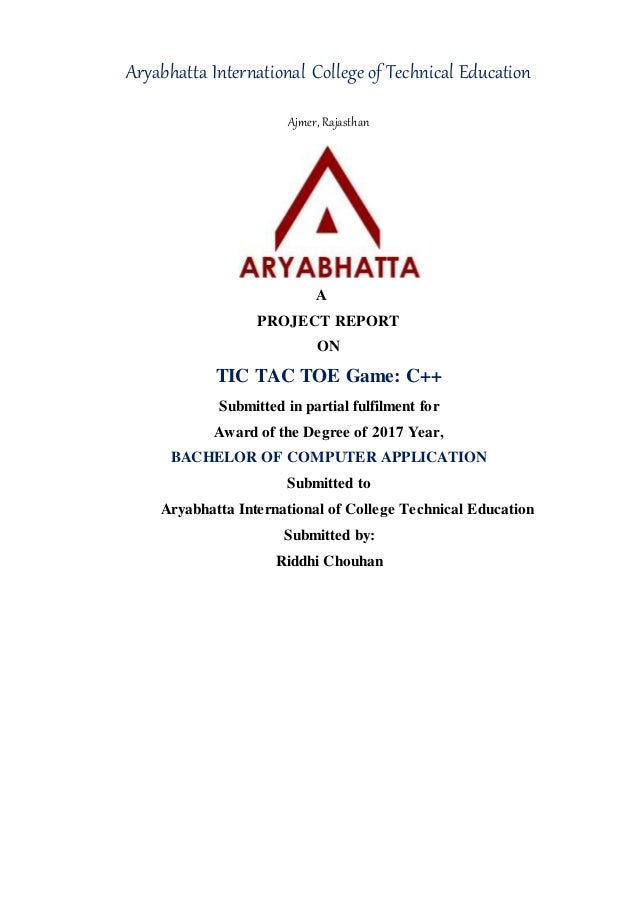Successfully reported this slideshow.
We use your LinkedIn profile and activity data to personalize ads and to show you more relevant ads. You can change your ad preferences anytime.Upcoming SlideShare
Loading in …5
×

# Tik tic tok

Tic-tac-toe (American English), noughts and crosses (British English), or Xs and Os is a paper-and-pencil game for two players, X and O, who take turns marking the spaces in a 3×3 grid. The player who succeeds in placing three of their marks in a horizontal, vertical, or diagonal row wins the game.

• Full Name
Comment goes here.

Are you sure you want to Yes No
Your message goes here• Login to see the comments

### Tik tic tok

1. 1. Aryabhatta International College of Technical Education Ajmer, Rajasthan A PROJECT REPORT ON TIC TAC TOE Game: C++ Submitted in partial fulfilment for Award of the Degree of 2017 Year, BACHELOR OF COMPUTER APPLICATION Submitted to Aryabhatta International of College Technical Education Submitted by: Riddhi Chouhan
2. 2. ACKNOWLEDGEMENT I am Riddhi Chouhan Student of Bachelor’s of computer application Aryabhatta International College Of Technical Education would like to express my gratitude to each and every person who has contributed in stimulating suggestions and encouragement which really helped me to co-ordinate my project. I also thank Aryabhatta International College Of Technical Education who provided insight and expertise that greatly assisted the project. Also, a special thanks to my teacher, parents and colleagues who have supported me at every step. Not to forget, the almighty who blessed me with good health becauseof which I worked more I worked more efficiently and better
3. 3. TIC TAC TOE Game : Start Game: Win Game:
4. 4. Draw Game: Code: #include<iostream.h> #include<conio.h> #include<stdlib.h> char square = {'o','1','2','3','4','5','6','7','8','9'}; int checkwin(); void board(); int main() { clrscr(); int player = 1,i,choice; char mark; do { board(); player=(player%2)?1:2;
5. 5. gotoxy(10,20); cout << "Player " << player << ", enter a number: "; cin >> choice; mark=(player == 1) ?'X' : '0'; if (choice == 1 && square == '1') square = mark; else if (choice == 2 && square == '2') square = mark; else if (choice == 3 && square == '3') square = mark; else if (choice == 4 && square == '4') square = mark; else if (choice == 5 && square == '5') square = mark; else if (choice == 6 && square == '6') square = mark; else if (choice == 7 && square == '7') square = mark; else if (choice == 8 && square == '8') square = mark; else if (choice == 9 && square == '9') square = mark; else
6. 6. { cout<<"Invalid move "; player--; cin.ignore(); cin.get(); } i=checkwin(); player++; }while(i==-1); board(); if(i==1) { gotoxy(35,24); cout<<"==>aPlayer "<<--player<<" win "; } else { gotoxy(35,24); cout<<"==>aGame draw"; } cin.ignore(); cin.get(); return 0; } int checkwin() { clrscr(); if (square == square && square == square)
7. 7. return 1; else if (square == square && square == square) return 1; else if (square == square && square == square) return 1; else if (square == square && square == square) return 1; else if (square == square && square == square) return 1; else if (square == square && square == square) return 1; else if (square == square && square == square) return 1; else if (square == square && square == square) return 1; else if (square != '1' && square != '2' && square != '3' && square != '4' && square != '5' && square != '6' && square != '7' && square != '8' && square != '9') return 0; else return -1; } void board() {
8. 8. int i; clrscr(); gotoxy(36,5); cout << "Tic Tac Toenn"; gotoxy(27,7); cout << "Player 1 (X) - Player 2 (O)"; gotoxy(33,10); cout << " " << square << " | " << square << " | " << square << endl; gotoxy(33,13); cout << " " << square << " | " << square << " | " << square << endl; gotoxy(33,16); cout << " " << square << " | " << square << " | " << square << endl; gotoxy(33,11); for(i=0;i<=17;i++) { cprintf("%c",220); } gotoxy(33,14); for(i=0;i<=17;i++) { cprintf("%c",220); } gotoxy(33,8); for(i=0;i<=17;i++) { cprintf("%c",220); } gotoxy(33,17); for(i=0;i<=17;i++) {
9. 9. cprintf("%c",220); } for(i=9;i<=17;i++) { gotoxy(32,i); cprintf("%c",219); } for(i=9;i<=17;i++) { gotoxy(50,i); cprintf("%c",219); } for(i=9;i<=17;i++) { gotoxy(38,i); cprintf("%c",219); } for(i=9;i<=17;i++) { gotoxy(44,i); cprintf("%c",219); } gotoxy(50,8); cprintf("%c",220); gotoxy(32,8); cprintf("%c",220); }
10. 10. Thank you Created By :-Riddhi Chouhan Aryabhatta International College of Technical Education